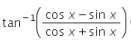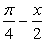Differentiatewith respect to x.a)-1b)c)π/4...

### Related TestDifferentiatewith respect to x.
• a)
-1
• b)• c)
π/4 − x
• d)
1Aadhar Academy(cosx − sinx)/(cosx + sinx) = (1 − tanx)/(1 + tanx)
tan(A − B) = (tanA − tanB)/(1 + tanAtanB)
= tan(π/4−x)
putting this value in question.
tan−1 tan(π/4−x)
π/4 − x.
so d(π/4 − x)/dx = -1View courses related to this question Explore JEE courses
 Explore JEE coursesView courses related to this question1 Crore+ students have signed up on EduRev. Have you?

• ### The beat frequency produced when the following two waves x1= 12 sin (484&pi... more(Scan QR code)